Libri di Igor Valli - libri Statistica & Applicazioni

# Igor Valli

### Author's titles

Decomposition by sources, by subpopulations and joint decomposition by subpopulations and sources of Gini, Bonferroni and Zenga 2007 inequality indexes digital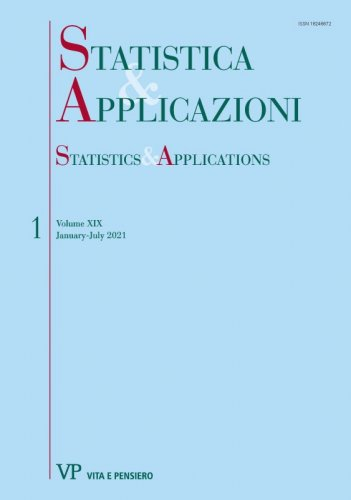Year: 2021
Recently, the authors have illustrated the decompositions by subpopulations of the Gini (1914), Bonferroni (1930) and Zenga (2007) inequality measures. These decompositions were illustrated by a numerical example involving non-overlapping subpopulations and by a numerical example involving overlapping subpopulations...
Decomposition by subpopulations of Gini, Bonferroni and Zenga inequality measures digital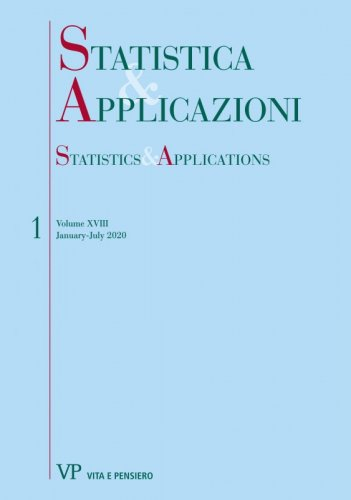Year: 2020
This paper presents a common framework for the decompositions by subpopulations of Gini, Bonferroni and Zenga synthetic inequality measures. These three synthetic indexes are the weighted arithmetic means of the corresponding point measures and applying the Zenga two-step approach, decompositions based on means comparison are obtained. In the first step additive decompositions are derived for the point indexes and in the second step, using the decompositions of the point measures, we obtain the decompositions by subpopulations of the synthetic indexes...
Joint decomposition by subpopulations and sources of the point and synthetic Gini indexes digital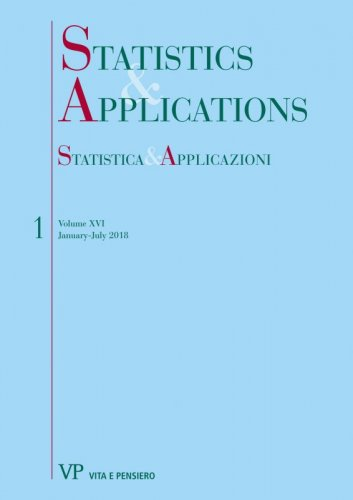Year: 2018
The decompositions proposed in this paper are applied to the net disposable income of the 8156 italian households supplied by Bank of Italy (2016) where the households are partitioned in four subpopulations according to the number of family members and the total income is the sum of four sources...
Joint decomposition by subpopulations and sources of the point and synthetic Bonferroni inequality measures digital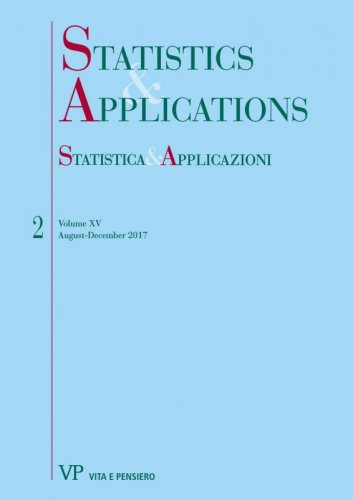Year: 2017
The total income Y is the sum of c sources Xj : Y = X1 + . . . + Xc: The N units of the population are partitioned in k different subpopulations. In the frequency distribution framework the Bonferroni (1930) point inequality index is given by Vh(Y) = [M(Y) - ̅Mh.(Y)]/M(Y), M(Y) and ̅Mh.(Y)are the mean and the lower mean of Y...
On the decomposition by subpopulations of the point and synthetic Bonferroni inequality measures digital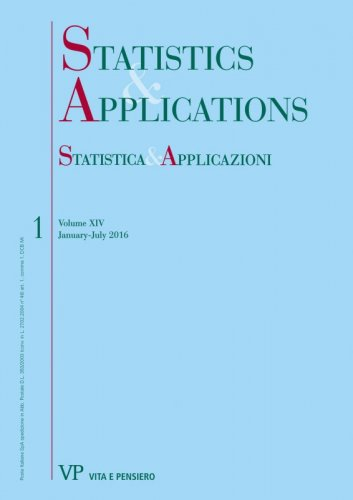Year: 2016
This paper, by using the ‘‘two-step’’ approach proposed in Radaelli (2008, 2010) and in Zenga (2016) for the decomposition of the Zenga (2007) index, obtains the decomposition of the Bonferroni (1930) inequality measure. In the first step the Bonferroni point measure Vh(Y) is decomposed in a weighted mean of k x k relative differences between the mean Mg(Y) of subpopulation g and the lower mean Mhl(Y) of the subpopulation l...